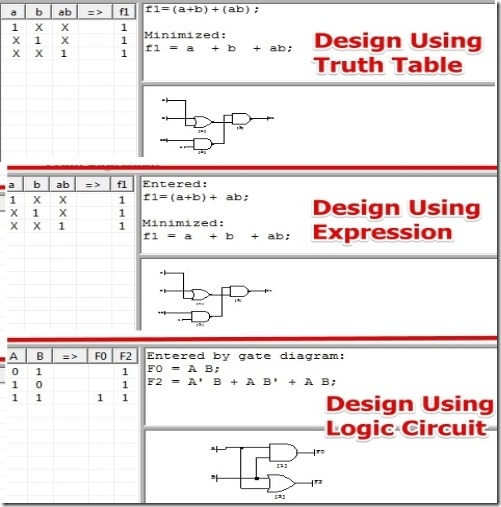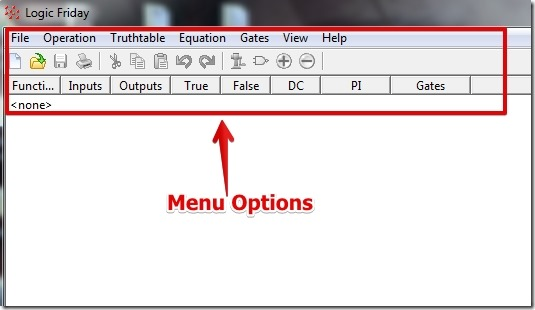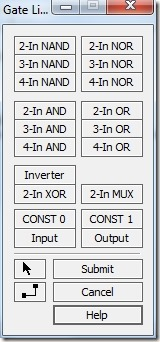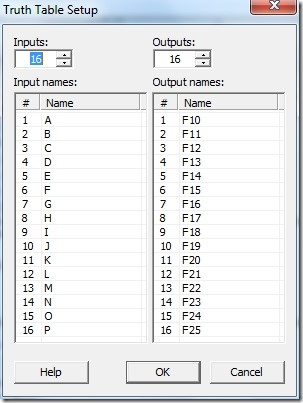Editor Ratings:
User Ratings:
[Total: 5 Average: 2.4]

Logic Friday is free software to design Logic Circuit, Truth Table, and Logic expression. With Logic Friday you require to know any one of the truth table, logic diagram, or the logical expression, you can create the other two unknown from one known expression.

Logic Friday lets you design logic circuit and you can convert the digital logic circuit into a truth table as well as the logic expression for the same.

Alternatively, you can write a logical expression and view the equivalent truth table and digital circuit. And, you can write a truth table and view the equivalent logical expression and logic circuit.

While using Logic Friday I really like that you can set the number of input and output for obtaining the digital circuit, set number of variables for the Truth Table, and select the number of outputs for the Logic Expression.## Using Logic Friday To Design Logic Circuit, Truth Table, and Logic Expression:

Logic Friday is very simple to use. When you launch Logic Friday you can select the option to start with a truth table, logic circuit, and logic expression under the file. You can select any options and input the required parameters (like truth table values, expression along with the output, or design a digital circuit) and then view the result.I have tested Logic Friday for all the three options and I was able to view the result easily.

For designing Digital Circuit: You can draw the digital circuit from the basic logic gates that you get. The logic gates include OR, AND , XOR, MUX, and CONSTANT. You have the option to use 2, 3 and 4 input gates which I really like.For creating a Truth Table: You can select this option and then edit the cells for 1, 0 and don’t care values for the output. I really like that when you map the truth table for the digital circuit you have the option to minimize the circuit and view the digital circuit for different logic gates.For writing a Logic Expression: You can simply write a logic expression, then view the result on the truth table and map the expression for the digital circuit diagram.

## Features of Logic Friday:

• Very effective and very fast calculation of result.
• Option to select Different number of input Gates.
• Minimize the logic expression.
• Don’t care values for the truth table.
• View Sum of Products, Product of Sum.
• Set functions for up to 16 different inputs and 16 outputs.
• Import and export truth tables as CSV files.
• Compare logic functions.

You might also like to try similar software Deeds.

## Conclusion:

Logic Friday offers a lot of options. I was able to design different Logic circuits, Truth Tables, and Logic Expressions very easily. Also, the option to simplify the equations and the option to set number of input and output for designing the logic circuit is really cool. I have had no problems using Logic Friday and it worked flawlessly for me. If you want to learn about Digital Circuits, Truth Tables, and Logic Expressions and why they represent each other, then you should try out Logic Friday.

Get Logic Friday Here.